Methods for Fit Model and Discover Key Predictors with TreeNet® Regression

Note

TreeNet® models are an approach to solving classification and regression problems that are both more accurate and resistant to overfitting than a single classification or regression tree. A broad, general description of the process is that we begin with a small regression tree as an initial model. From that tree come residuals for every row in the data which become the response variable for the next regression tree. We build another small regression tree to predict the residuals from the first tree and compute the resulting residuals again. We repeat this sequence until an optimal number of trees with minimum prediction error is identified using a validation method. The resulting sequence of trees makes the TreeNet® Regression Model.

For the regression case, we can add a general description of the analysis, but some details depend on which of the following is the loss function:

Table 1. Values for the squared error loss function
Statistic Value
Initial fit,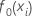mean of the response variable
Generalized residual,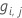as response value for row i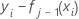Within node updates,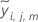mean ofTable 2. Values for the absolute deviation loss function
Statistic Value
Initial fit,median of the response variable
Generalized residual,as response value for row i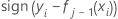Within node updates,median ofHuber loss function

For the Huber loss function, the statistics are as follows:

The initial fit,, equals the median of all response values.

For growing the jth tree,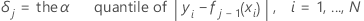Then, the generalized residual for the ith row is as follows: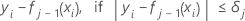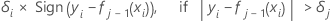The generalized residuals are used as the response values to grow the jth tree.

The updated value for rows in the mth terminal node of the jth tree is as follows:

Define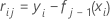to be the regular residual for the ith row after j-1 trees are grown. Let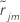be the median of the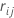values for rows inside terminal node m of the jth tree. Then, the updated value for every row inside the mth terminal node of the jth tree is:

The mean in the previous expression is calculated across all the rows inside the terminal node m of the jth tree.

Notation for loss functions

In the preceding details,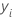is the value of the response variable for row i,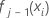is the fitted value from the previous j – 1 trees, and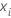is a vector that represents the ith row of the predictor values in the training data.

Input parameters

The model creation also uses the following inputs from the analyst:
Input Symbol
learn rate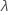sampling ratemaximum number of terminal nodes per tree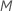number of treesswitching value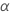General process

The process has the following general steps for growing the jth tree, j = 1, ..., J:
1. Draw a random sample of size s * N from the training data, where N is the number of rows in the training data.
2. Calculate the generalized residuals,, for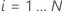.
3. Fit a regression tree with at most M terminal nodes to the generalized residuals. The tree partitions the observations into at most M mutually exclusive groups.
4. For the mth terminal node in the regression tree, calculate the within-node updates to the tree that depend on the loss function,.
5. Shrink the within-node updates by the learning rate and apply the values to get the updated fitted values,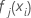:
6. Repeat steps 1-5 for each of the J trees in the analysis.
By using this site you agree to the use of cookies for analytics and personalized content.  Read our policy